×#### Thank you for registering.

One of our academic counsellors will contact you within 1 working day.

Click to Chat

1800-1023-196

+91-120-4616500

CART 0

• 0

MY CART (5)

Use Coupon: CART20 and get 20% off on all online Study Material

ITEM
DETAILS
MRP
DISCOUNT
FINAL PRICE
Total Price: Rs.

There are no items in this cart.
Continue Shopping• Complete JEE Main/Advanced Course and Test Series
• OFFERED PRICE: Rs. 15,900
• View Details

```Chapter 3: Squares and Square Roots Exercise – 3.6

Question: 1

Find the square root of: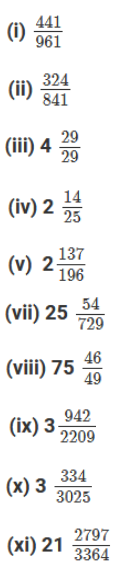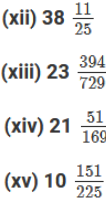Solution: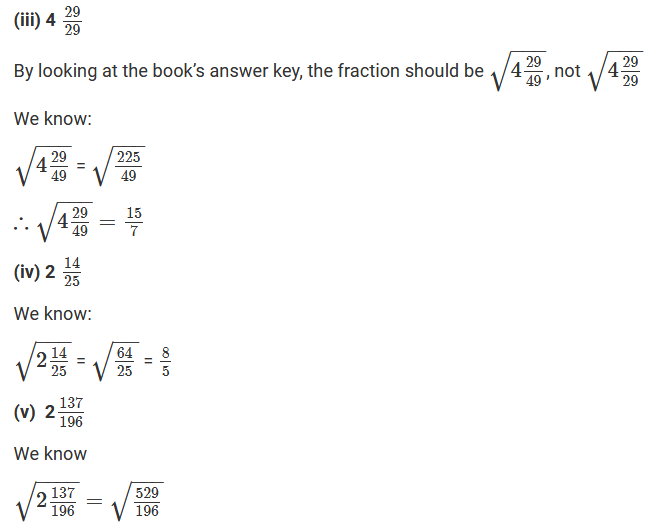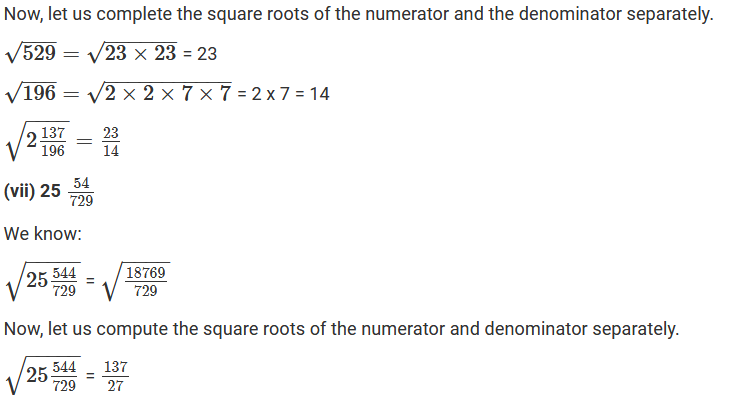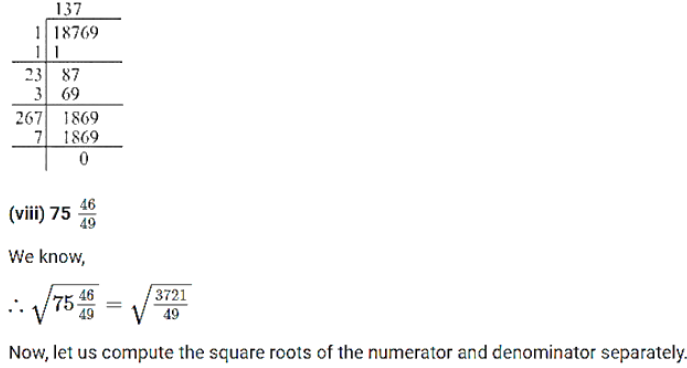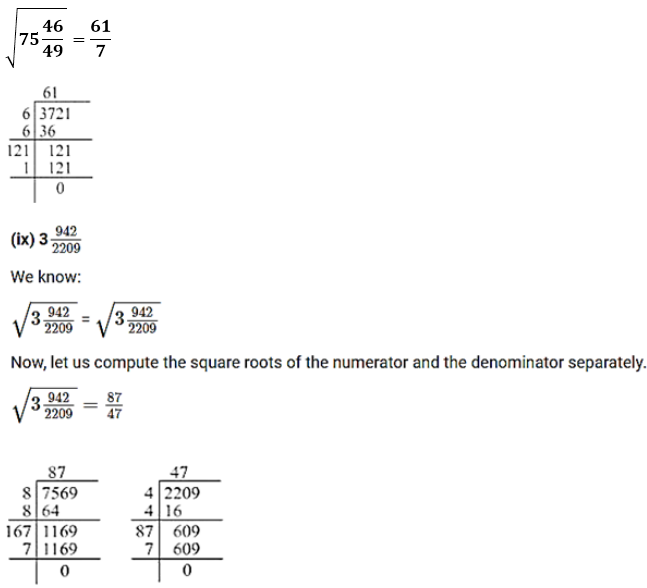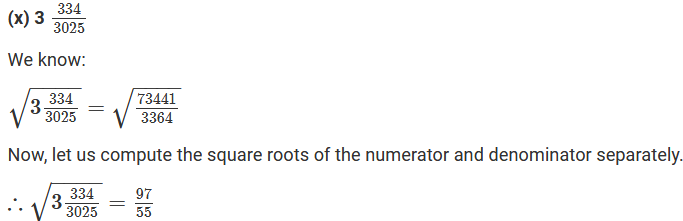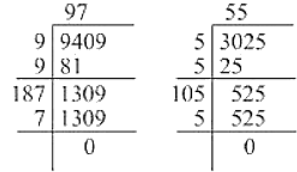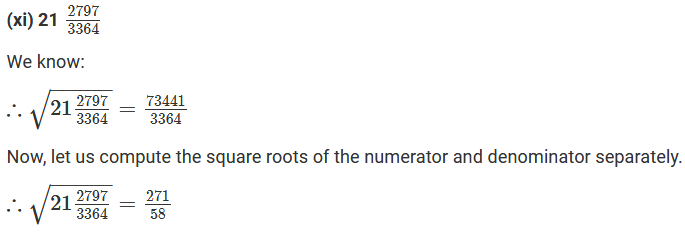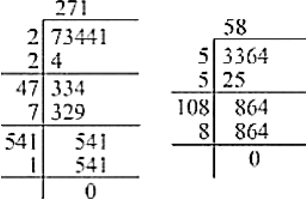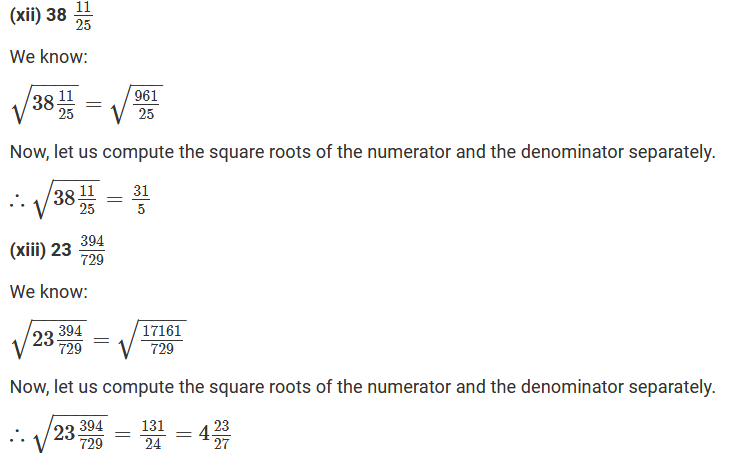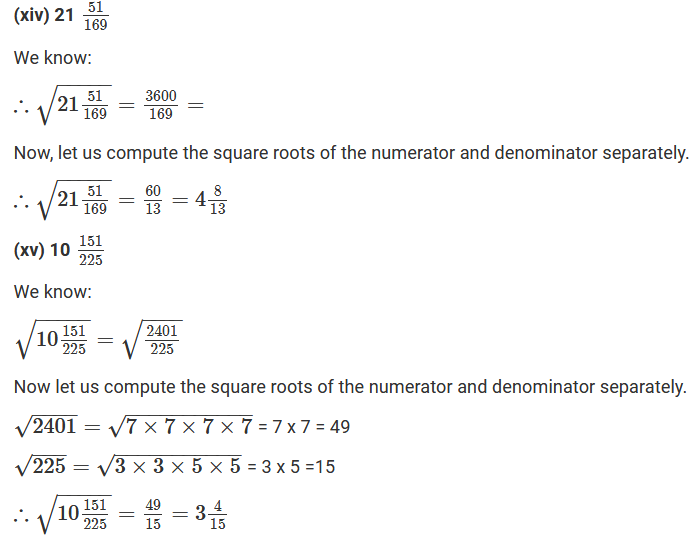Question: 2

Find the value of: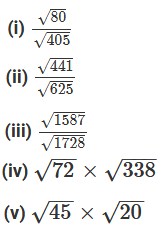Solution: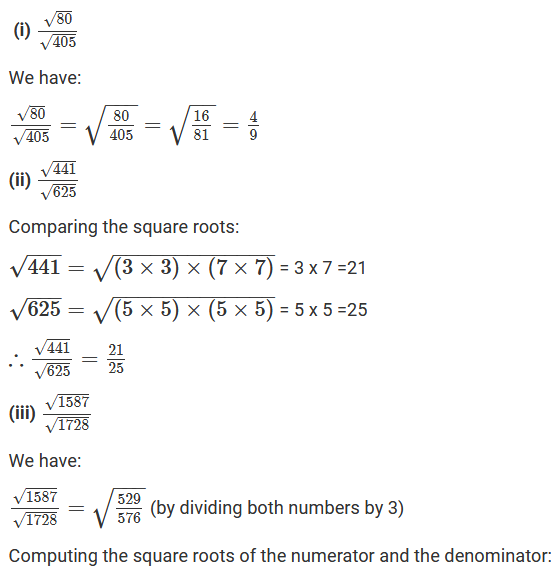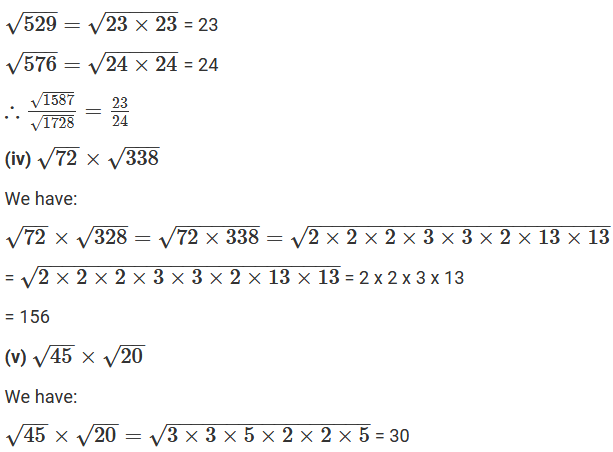Question: 3

The area of a square is 80 244/729 square metres. Find the length of each side of the field.

Solution: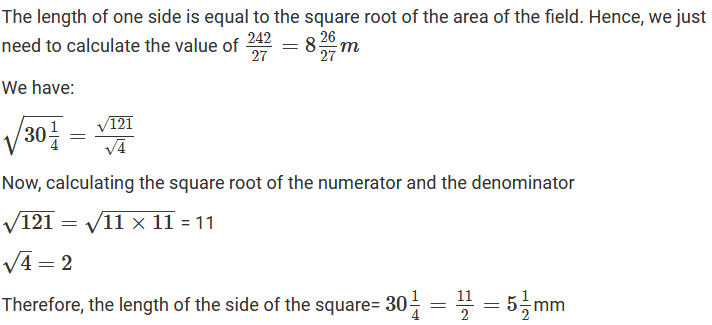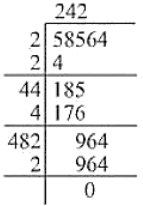Question: 4

The area of a square field is 30(1/4) m2. Calculate the length of the side of the square.

Solution:Question: 5

Find the length of a side of a square playground whose area is equal to the area of a rectangular field of dimensions 72 m and 338 m.

Solution:

The area of the playground = 72 x 338 = 24336 m2

The length of one side of a square is equal to the square root of its area. Hence, we just need to find the square root of 24336.

Hence, the length of one side of the playground is 156 meters.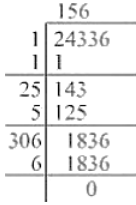```### Course Features

• 728 Video Lectures
• Revision Notes
• Previous Year Papers
• Mind Map
• Study Planner
• NCERT Solutions
• Discussion Forum
• Test paper with Video Solution